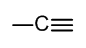# 2.3: How to judge hybridization of an atom

$$\newcommand{\vecs}{\overset { \rightharpoonup} {\mathbf{#1}} }$$ $$\newcommand{\vecd}{\overset{-\!-\!\rightharpoonup}{\vphantom{a}\smash {#1}}}$$$$\newcommand{\id}{\mathrm{id}}$$ $$\newcommand{\Span}{\mathrm{span}}$$ $$\newcommand{\kernel}{\mathrm{null}\,}$$ $$\newcommand{\range}{\mathrm{range}\,}$$ $$\newcommand{\RealPart}{\mathrm{Re}}$$ $$\newcommand{\ImaginaryPart}{\mathrm{Im}}$$ $$\newcommand{\Argument}{\mathrm{Arg}}$$ $$\newcommand{\norm}{\| #1 \|}$$ $$\newcommand{\inner}{\langle #1, #2 \rangle}$$ $$\newcommand{\Span}{\mathrm{span}}$$ $$\newcommand{\id}{\mathrm{id}}$$ $$\newcommand{\Span}{\mathrm{span}}$$ $$\newcommand{\kernel}{\mathrm{null}\,}$$ $$\newcommand{\range}{\mathrm{range}\,}$$ $$\newcommand{\RealPart}{\mathrm{Re}}$$ $$\newcommand{\ImaginaryPart}{\mathrm{Im}}$$ $$\newcommand{\Argument}{\mathrm{Arg}}$$ $$\newcommand{\norm}{\| #1 \|}$$ $$\newcommand{\inner}{\langle #1, #2 \rangle}$$ $$\newcommand{\Span}{\mathrm{span}}$$$$\newcommand{\AA}{\unicode[.8,0]{x212B}}$$

### Hybridization

Hybridization is a simple model that deals with mixing orbitals to from new, hybridized, orbitals. This is part of the valence bond theory and helps explain bonds formed, the length of bonds, and bond energies; however, this does not explain molecular geometry very well.

• sp  An example of this is acetylene (C2H2). This combines one s orbital with one p orbital. This means that the s and p characteristics are equal.
• sp2 An example of this is ethylene (C2H4). This is the combination of one s orbital and two p orbitals.
• sp3 An example of this is methane (CH4). This is the combination of one s orbital and three p orbitals.

If you add the exponents of the hybridized orbitals, you get the amount of sigma bonds associated with that bond. The sp2 hybridized orbital has one p orbitals that is not hybridized and so it can form a pi bond. This means that sp2 orbitals allow for the formation of a double bond. Also, sp hybridized orbitals form a triple bond.

The hybrid orbitals used (and hence the hybridization) depends on how many electron groups are around the atom in question.  An electron group can mean either a bonded atom or a lone pair.  Examples are shown for carbon; nitrogen and oxygen are similar, with lone pairs taking the place of single bonds:

 No. of electron groups Hybrid orbital used Example Bond angle 2 sp180o 3 sp2120o 4 sp3109.5o

However, there is an even easier way to judge the hybridization:

### SIMPLE WAY to work out hybridization

This is a simple way to find out the hybridization for an atom of carbon, nitrogen or oxygen.

• All single bonds or lone pairs => sp3
• One double bond => sp2
• One triple bond => sp

### Example

Look at the structure of thiamine diphosphate in the ‘structures of common coenzymes’ table.  Identify the hybridization of all carbon atoms in the molecule.### Useful resources